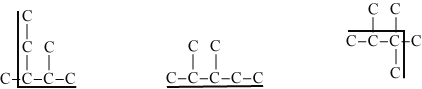Time Limit : sec, Memory Limit : KB
English

# Problem C: Finding the Largest Carbon Compound Given Its Longest Chain

An bydrocarbon is an organic compound which contains only carbons and hydrogens. An isomer is a compound that has the same number of carbons but different structures. Heptane, for example, is a hydrocarbon with 7 carbons. It has nine isomers. The structural formula of three are shown in Figure 1. Carbons are represented by the letter C, and bonds between carbons are represented by a straight line. In all figures, hydrogens are not represented for simplicity. Each carbon can be connected to a maximum of 4 carbons.Figure 1: These three examples of isomers of heptane have the same number of carbons but different structures.

Let define a chain in an isomer as a sequence of connected carbons without branches. An isomer can have many chains of the same length. Figure 2 shows the longest chain of carbons for each of the represented isomer. Note that there can be many instances of longest chain in an isomer.Figure 2: The figures shows one instance of longest chain of carbons in each isomer. The first and the second isomers show longest chains of 5 carbons. The longest chain in the third isomer has 4 carbons.

Your task is to identify the number of carbons of the largest possible carbon compound whose longest carbon chain has n (1 ≤ n ≤ 30) carbons.

## Input

Each input contains a list of number of carbons, i.e. the length of a carbon chain.

The number of input lines is less than or equal to 30.

## Output

For each number n in input, identify the number of carbons of the largest possible carbon compound whose longest carbon chain has n carbons.

## Sample Input

1
4


## Output for the Sample Input

1
8